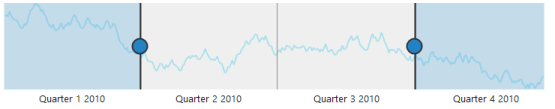# Populate Data

17 Nov 20172 minutes to read

When you provide data to RangeNavigator, it produces limited set of data. You can populate the RangeNavigator with data using the `DataSource` and `Series` properties.

## Add series to the RangeNavigator

The `Series` property provides access to a collection of all series that are defined explicitly within a RangeNavigator control. Each series is assigned with `Type` and `Name`. It contains collection of data point, each point contains x value and y values. You can add data points to the series through `DataSource` property field name to get the values from the dataSource in `XName` and `YName` options.

Animation can be enabled by setting `EnableAnimation` property as true and the series color and opacity can be customized by using `Fill` and `Opacity` property in series.

• CSHTML
• ``````@(Html.EJ().RangeNavigator("container")

.Series(sr =>

{

sr.Name("ProductA")

.EnableAnimation(false)

.Type(SeriesType.Line)

.Opacity(0.5)

})

<script type="text/javascript">

{

data = GetData();

sender.model.series.dataSource = data.Open;

sender.model.series.xName =  "XValue",

sender.model.series.yName = "YValue";

}

// Method to get data in JSON format

function GetData()
{

var series1 = [];

var series2 = [];

var value = 100;

var value1 = 120;

for (var i = 1; i < 730; i++)
{

if (Math.random() > .5)
{

value += Math.random();

value1 += Math.random();

}
else
{

value -= Math.random();

value1 -= Math.random();

}

var point1 = { XValue: new Date(2010, 0, i), YValue: value };

var point2 = { XValue: new Date(2010, 0, i), YValue: value1 };

series1.push(point1);

series2.push(point2);

}

data = { Open: series1, Close: series2 };

return data;

}

</script>``````

The following screenshot illustrates the RangeNavigator that is populated with data using DataSource property in series.